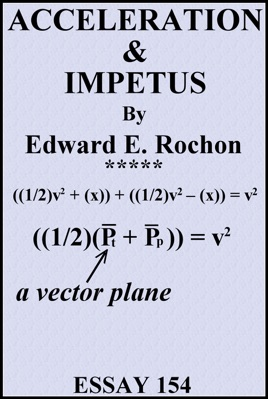• USD 1.99

## Descripción de editorial

A preface introduces the topic and briefly mentions Isaac Newton and Madame du Châtelet. Chapter 1 previews the problem. Newton thought that acceleration and momentum (or energy within a moving object was proportional. Leibniz suggested and Châtelet tested the thesis that it varied by the square. Leibniz proved correct in his conjecture. Chapter 2 gives my explanation of why this is the square, rather than the cube, for example, since energy must distribute into a volume or cube function. We use vector planes and the concept of inertia as impetus to explain the geometric square relationship.